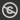Science, Maths & Technology
Free course

# Group theory## Course reviews

This free course consolidates and builds on group theory studied at OU level 2 or equivalent. Section 1 describes how to construct a group called the direct product of two given groups, and then describes certain conditions under which a group can be regarded as the direct product of its subgroups. Section 2 describes the key properties of the structure of cyclic groups. Section 3 introduces the notion of a set of generators of a group and a set of relations among the generators. It then looks at a family of finite groups, the dicyclic groups.

## Course learning outcomes

After studying this course, you should be able to:

• apply the Internal Direct Product Theorem in simple cases
• decide whether a given group is cyclic, and given a finite cyclic group, find a generator for a subgroup of a given order
• express a given finite cyclic group as the direct product of cyclic groups of prime power order and, given two direct products of cyclic groups, determine whether or not they are isomorphic
• express products of elements of a group defined by generators and relations in appropriate standard form
• recognise the dihedral and dicyclic groups when described using a standard form.

First Published: 09/03/2018

Updated: 07/12/2018

Skip Rate and Review